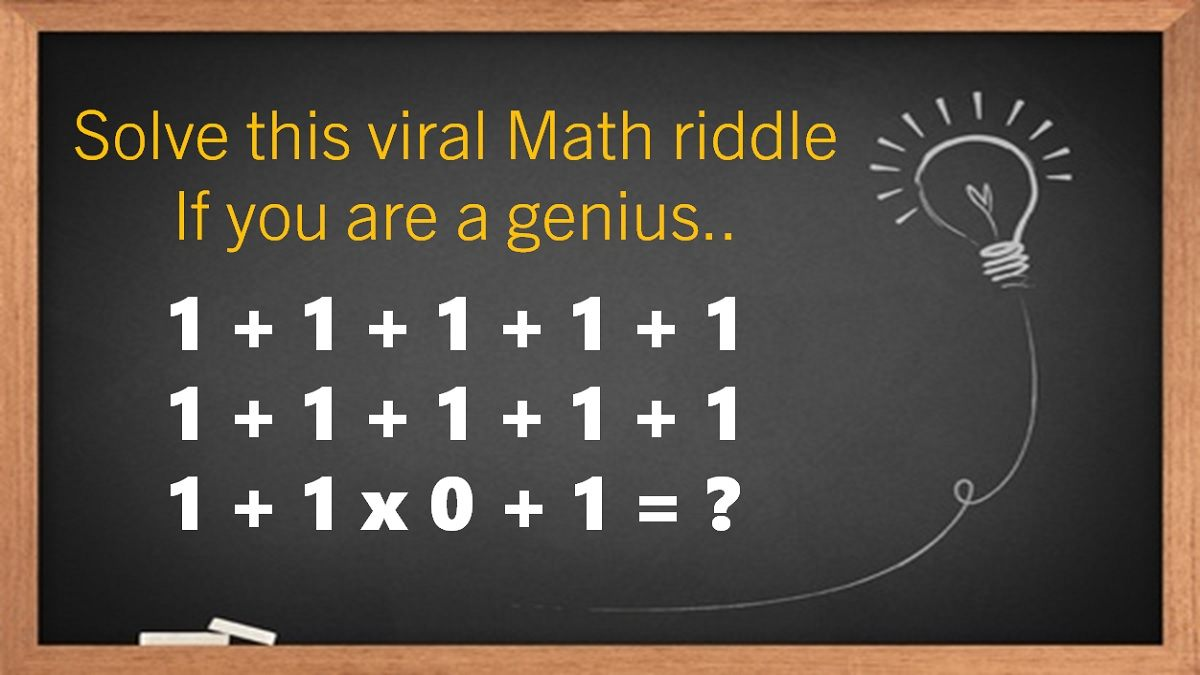# Math Riddles: Only High IQ Genius Can Solve These

Math Riddles with Answers: Can you solve these tricky math riddles? Only 2% of people with a high IQ can get the answer right. Check out these math riddles for geniuses!Math Riddles Only Genius Can Solve These

Math Riddles are so challenging, but that makes them worthwhile to solve. Math riddles are logical problems that require strong analytical abilities, high IQ, knowledge of math concepts, and good calculations skills. Math riddles have also been known to make Mathematics enjoyable for kids, students, and even adults to solve. Riddles, puzzles, and brain teasers can develop strategic thinking, logical reasoning, and problem-solving skills.

## Math Riddle #5

Also Read: Math Riddles with Answers: Are you Genius? Find All Missing Numbers

1 + 1 + 1 + 1 + 1

1 + 1 + 1 + 1 + 1

1 + 1 x 0 + 1 =??

We apply the BODMAS rule (B – Brackets, O – Order of powers or roots, D – Division, M – Multiplication A – Addition, and S – Subtraction).

First, multiply the 1 x 0 in the third line which gives 0.

1 + 1 + 1 + 1 + 1

1 + 1 + 1 + 1 + 1

1 + 0 + 1

Now, before we start adding up all the 1s, we notice that the last 1 in the first line as well as the second line do not have any operation such as (+/-/x/÷) next to them.

So, we have to assume that the last 1 in the first line and the first 1 in the second line form 11 which will give us this equation: 1+1+1+1+11

Moving on, we have 1 + 1 + 1 in the second line and again the 1 at the end in the second line with no operation next to it. So, we will again assume that the last 1 of the second line and the first 1 of the third line form 11. Now, we have the following equation below:

1+1+1+1+11+1+1+1+11+0+1 which equals to 30.

If 4+2=26

8+1=79

6+5=111

Then, what is 7+3=??

4+2=26 is because 4-2=2 and 4+2=6, so it is 26. Similarly, 8+1=79 because 8-1=7 and 8+1=9, so it is 79, and 6+5=111 because 6-5=1 and 6+5=11, so it 111.

Therefore, 7+3 will be 410 because 7-3=4 and 7+3=10, so it will be 410.

If you multiply all the numbers on the telephone number pad…

What number do you get?

The number is Zero (0).

The numbers on a telephone's number pad are 0–9. So multiplying them all together gives 0, because anything multiplied by 0 is 0.

7 + 7 ÷ 7 + 7 x 7 – 7 =??

We shall apply the BODMAS rule:

Step 1: Divide the numbers 7 ÷ 7

7 + 1 + 7 x 7 - 7

Step 2: Multiply the numbers 7 x 7

7 + 1 + 49 - 7

Step 3: Addition of numbers 7 + 1

8 + 49 - 7

Step 4: Subtracting the remaining 7

57 – 7 = 50

If seven people meet each other and each shakes hands only once with each of the others.

How many handshakes will be there in total?

Most people would think there were 42 handshakes. The first person shakes the hand of 6 others, the second person shakes the hand of 5 remaining people, the third person shakes the hand of 4 remaining people, the fourth person shakes the hand of 3 remaining people, and the 5th person shakes the hand of 2 remaining people, and the sixth person shakes the hand of 1 remaining person. 6+5+4+3+2+1=21

## Tell us in comments: Did you solve all these tricky math riddles?

Also Read: Math Riddles with Answers: Only the 1% Smartest Can Find All Missing Numbers

Also Read: Tricky IQ Riddles: Only 1 in 5 people with high IQ can solve these

Also Read: Personality Test: 5 Signature Styles Reveal These Personality Traits